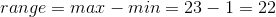Example Questions

2 Next →

Example Question #11 : Range

Mrs. Jung gave a geometry test to her 15 students. The scores were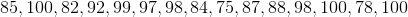. What is the range of the test scores?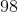Explanation:

The range of scores is from 75 to 100, which is a range of 25 points.

You take the highest score and subtract the lowest score from it.

The highest score is 100 and the lowest score is 75 therefore the range is as follows.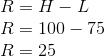Example Question #12 : Range

Adam is comparing the prices of granola at different grocery stores in town. So far, these are the prices he's found: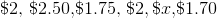If the average of these prices is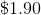then what is the range of all the prices?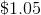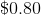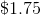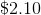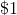Explanation:

First, we want to solve for x. Since we know the average of all the prices is \$1.90, then we can say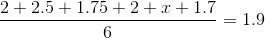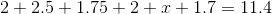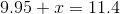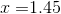Now, we can find the range of the prices. The range is represented by the highest value minus the lowest value in any set. With that in mind, our range is given by: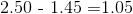Example Question #13 : Range

A word game comprises 100 square tiles, each of which has a letter and a numeric value. Sixty of the tiles have value 1 each; twenty tiles have value 2 each; twelve of the tiles have value 4 each; four tiles have value 8 each; and two tiles have value 10 each. There are also two blank tiles with zero value.

What is the mean of the values of the tiles?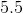None of the other responses is correct.Explanation:

The total value of the tiles is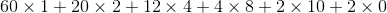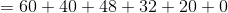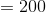There are 100 tiles, so the mean value is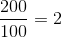Example Question #14 : Range

Find the range of the following set of numbers:

1,5,14,17,22,23,23